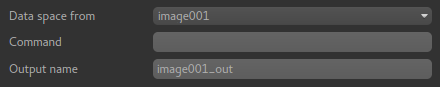# Simple maths¶

Widgets -> Processing -> Simple Maths

This widget is a simplified version of the console and allows new data to be created from simple operations on existing data.The data space for output must be specified by selecting a data set - this is necessary because it’s not generally possible to analyse the expression and determine the output space. Usualy the output data space will match the data space of the data sets used in the input.

The Command text entered must be a valid Python expression and can include the names of existing ROIs and overlays which will be Numpy arrays. Numpy functions can be accessed using the np namespace. Some knowledge of the Numpy library is generally needed to use this widget effectively.

An output name for the data set is also required.

## Examples¶

Add Gaussian noise to some data:

mydata + np.random.normal(0, 100)


Calculate the difference between two data sets:

mydata1 - mydata2


Scale data to range 0-1:

(mydata - mydata.min()) / (mydata.max() - mydata.min())